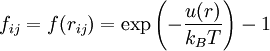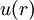# Mayer f-function$f_{ij}=f(r_{ij})= \exp\left(-\frac{u(r)}{k_BT}\right) -1$
•$k_B$ is the Boltzmann constant
•$T$ is the temperature
•$u(r)$ is the potential